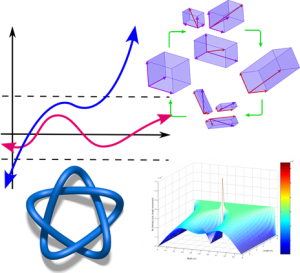Masks are required as the campus is at red status.

# Mathematics & Statistics MinorsThe department of mathematics offers two options for a mathematics minor, and one option for a statistics minor. Adding one of these minors to your degree can enhance your expertise and create a solid foundation in quantitative science. Contact Ramesh Garimella or any of the math department faculty about adding a mathematics minor to your degree.

 Minor in Mathematics (Option 1) Required Courses Course Number Course Credit Hours Prerequisite Semester Offered MATH 1496 Calculus I 4 C or better in MATH 1390 and MATH 1392; or C or better in MATH 1580; or equivalent Fall, Spring, & Summer MATH 1497 Calculus II 4 C or better in MATH 1496 Fall, Spring, & Summer MATH 2335 Transition to Advanced Math 3 MATH 1497 Fall & Spring MATH 3360 Rings and Fields 3 MATH 2335 Fall Elective Courses: Choose 1 of these MATH 2471 Calculus III 4 C or better in MATH 1497 Fall, Spring, & Summer MATH 3320 Linear Algebra [Upper Core: I] 3 MATH 1497 or MATH 2330 Fall, Spring, & Summer MATH 3331 Ordinary Differential Equations I [Upper Core : C] 3 MATH 1497 Fall, Spring MATH 4340 Numerical Methods 3 C or better in both MATH 3320 and MATH 2441 Fall MATH 3362 Introduction to Group Theory 3 MATH 2335 Spring MATH 4371 Introduction to Probability [Upper Core: R] 3 MATH 1497 Fall MATH 4375 Introduction to Topology I 3 Consent of instructor On Demand MATH 4385 Complex Analysis 3 MATH 2471 On Demand

 Minor in Mathematics (Option 2) Required Courses Course Number Course Credit Hours Prerequisite Semester Offered MATH 1496 Calculus I 4 C or better in MATH 1390 and MATH 1392; or C or better in MATH 1580; or equivalent Fall, Spring, & Summer MATH 1497 Calculus II 4 C or better in MATH 1496 Fall, Spring, & Summer MATH 3320 Linear Algebra [Upper Core: I] 3 MATH 1497 or MATH 2330 Fall, Spring, & Summer Elective Courses: Choose 2 of these MATH 2471 Calculus III 4 C or better in MATH 1497 Fall, Spring, & Summer MATH 3331 Ordinary Differential Equations I [Upper Core : C] 3 MATH 1497 Fall, Spring MATH 3360 Rings and Fields 3 MATH 2335 Fall MATH 3362 Introduction to Group Theory 3 MATH 2335 Spring MATH 4305 Ordinary Differential Equations II 3 MATH 3320 and MATH 3331 Fall MATH 4340 Numerical Methods 3 C or better in both MATH 3320 and MATH 2441 Fall MATH 4371 Introduction to Probability [Upper Core: R] 3 MATH 1497 Fall MATH 4375 Introduction to Topology I 3 Consent of instructor On Demand MATH 4385 Complex Analysis 3 MATH 2471 On Demand

 Minor in Statistics Required Courses Course Number Course Credit Hours Prerequisite Semester Offered MATH 2311 Statistics I 3 MATH 1390 or equivalent Fall, Spring, & Summer MATH 3311 Statistics II 3 MATH 2441 or CSCI 1496 & 1491, or consent of instructor Fall, Spring & Summer MATH 3320 Linear Algebra [Upper Core: I] 3 MATH 1497 or MATH 2330 Fall, Spring, & Summer MATH 4371 Introduction to Probability [Upper Core: R] 3 MATH 1497 Fall MATH 4372 Introduction to Statistical Inference 3 MATH 2441 and 4371 Spring MATH 4373 Regression Analysis 3 MATH 3311 (C or higher) or consent of instructor Fall

Can’t decide what to take? Ask a professor in the math department. Or if you want an exposure to the traditional pure math courses take 1496, 1497, 2335, 3360, and 3362. For an exposure to the traditional applied math courses, take 1496, 1497, 2471, 3320, and 3331.

Version Fall 2018